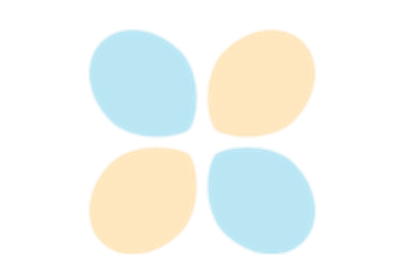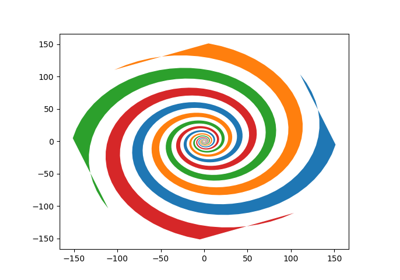You are reading an old version of the documentation (v2.2.3). For the latest version see https://matplotlib.org/stable/api/_as_gen/matplotlib.pyplot.fill.html

# matplotlib.pyplot.fill¶

`matplotlib.pyplot.``fill`(*args, **kwargs)[source]

Plot filled polygons.

Parameters: args : sequence of x, y, [color] Each polygon is defined by the lists of x and y positions of its nodes, optionally followed by by a color specifier. See `matplotlib.colors` for supported color specifiers. The standard color cycle is used for polygons without a color specifier. You can plot multiple polygons by providing multiple x, y, [color] groups. For example, each of the following is legal: ```ax.fill(x, y) # a polygon with default color ax.fill(x, y, "b") # a blue polygon ax.fill(x, y, x2, y2) # two polygons ax.fill(x, y, "b", x2, y2, "r") # a blue and a red polygon ``` a list of :class:`~matplotlib.patches.Polygon` **kwargs : `Polygon` properties

Notes

Use `fill_between()` if you would like to fill the region between two curves.

Note

In addition to the above described arguments, this function can take a data keyword argument. If such a data argument is given, the following arguments are replaced by data[<arg>]:

• All arguments with the following names: 'x', 'y'.

## Examples using `matplotlib.pyplot.fill`¶Interactive functionsFill Spiral